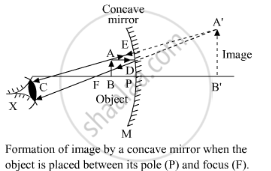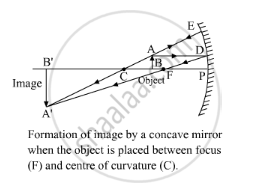Share

# Give Two Circumstances in Which a Concave Mirror Can Form a Magnified Image of an Object Placed in Front of It. Illustrate Your Answer by Drawing Labelled Ray Diagrams for Both. - CBSE Class 10 - Science

ConceptMirror Formula and Magnification

#### Question

Give two circumstances in which a concave mirror can form a magnified image of an object placed in front of it. Illustrate your answer by drawing labelled ray diagrams for both.

#### Solution

The two circumstances in which a concave mirror can form a magnified image of an object that is placed in front of it are:

1. When an object is placed between the pole and the focus of a concave mirror, the image formed is virtual, erect and magnified, as shown in the figure. Here, the object AB is placed between the pole and the focus of a concave mirror. Its image A'B' is formed behind themirror and is virtual, erect and magnified.

1. When an object is placed between the centre of curvature and the focus of a concave mirror, the image formed is real, inverted and enlarged, as shown in figure 2. Here, the object AB is placed between the centre of curvature and the focus of a concave mirror. Its image A'B' is formed beyond the centre of curvature and is real, inverted and enlarged.Is there an error in this question or solution?

#### Video TutorialsVIEW ALL 

Solution Give Two Circumstances in Which a Concave Mirror Can Form a Magnified Image of an Object Placed in Front of It. Illustrate Your Answer by Drawing Labelled Ray Diagrams for Both. Concept: Mirror Formula and Magnification.
S